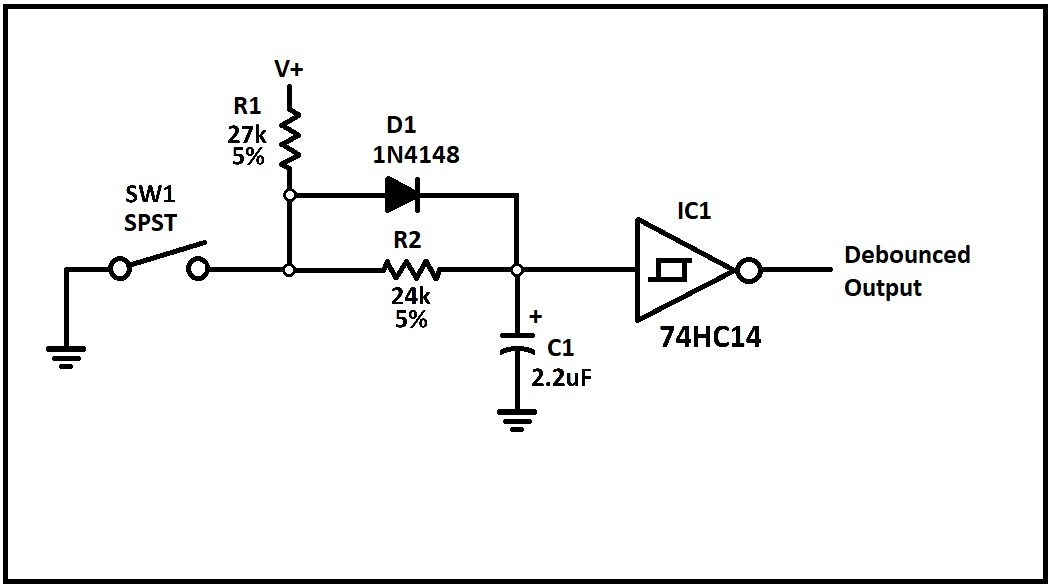# METHOD 4. HARDWARE DEBOUNCE FOR SPST SWITCHES.There are two best methods for debouncing high-speed circuits where the simpler single pole-single throw (SPST) switches are used.

1. Hardware SPST Debounce

2. Software SPST Debounce

Whenever a single pole-single throw (SPST) switch is used for input into high-speed digital devices, the only option is to wait out the bounce interval with either hardware or software delays (see Waveform 2 below). Both the hardware and software methods simply delay the switch output until after the bounce time has ended. The individual application dictates the usefulness of this debounce strategy. Most applications can handle delays of up to 100 milliseconds with a barely noticeable delay.

This circuit charges the capacitor C1 through Resistor R1 and Diode D1 when the switch is released, and discharges it through Resistor R2 when it is activated. The 74HC14 Schmitt trigger IC provides the needed hysteresis for a snap-action output when the slow ramp of the RC circuit rises and falls. Hysteresis assures a single transition with no oscillation when the switch is activated or released.

NOTE: The values shown in the drawing provide delays of approximately 36 milliseconds on activation and release with a 5 volt supply. The resistance of R1 and R2 may be raised or lowered for longer or shorter delay times.

Using a 2.2uF Capacitor for C1, a very simple way to calculate the delay time for the circuit shown is R1 = T * 750, and R2 = R1 * 0.9

where T is the desired delay time in milliseconds, and  R1 & R2 are resistance in ohms:

For 100ms delay:    R1 = 100 * 750              R1 = 75,000 ohms

R2 = R1 * 0.9                 R2 = 75,000 * 0.9            R2 = 67,500 ohms

For 20ms delay:      R1 = 20 * 750                R1 = 15,000 ohms

R2 = R1 * 0.9                 R2 = 15,000 * .9              R2 = 13,500 ohms